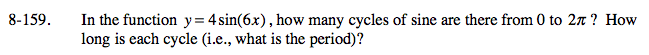Home > A2C > Chapter 8 > Lesson 8.2.4 > Problem8-159

8-159.

In the function y = 4sin(6x), how many cycles of sine are there from 0 to 2π? How long is each cycle (i.e., what is the period)? Homework Help ✎Refer to the Math Notes box for this lesson.

The period is equal to 2π divided by the number of cycles from 0 to 2π.